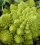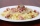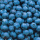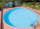# Fraction calculator

The calculator performs basic and advanced operations with fractions, expressions with fractions combined with integers, decimals, and mixed numbers. It also shows detailed step-by-step information about the fraction calculation procedure. Solve problems with two, three, or more fractions and numbers in one expression.

## Result:

### (52/3) : 4 = 17/12 = 1 5/12 ≅ 1.4166667

Spelled result in words is seventeen twelfths (or one and five twelfths).

### How do you solve fractions step by step?

1. Conversion a mixed number 5 2/3 to a improper fraction: 5 2/3 = 5 2/3 = 5 · 3 + 2/3 = 15 + 2/3 = 17/3

To find a new numerator:
a) Multiply the whole number 5 by the denominator 3. Whole number 5 equally 5 * 3/3 = 15/3
b) Add the answer from previous step 15 to the numerator 2. New numerator is 15 + 2 = 17
c) Write a previous answer (new numerator 17) over the denominator 3.

Five and two thirds is seventeen thirds
2. Divide: 17/3 : 4 = 17/3 · 1/4 = 17 · 1/3 · 4 = 17/12
Dividing two fractions is the same as multiplying the first fraction by the reciprocal value of the second fraction. The first sub-step is to find the reciprocal (reverse the numerator and denominator, reciprocal of 4/1 is 1/4) of the second fraction. Next, multiply the two numerators. Then, multiply the two denominators. In the following intermediate step, it cannot further simplify the fraction result by canceling.
In other words - seventeen thirds divided by four = seventeen twelfths.

#### Rules for expressions with fractions:

Fractions - simply use a forward slash between the numerator and denominator, i.e., for five-hundredths, enter 5/100. If you are using mixed numbers, be sure to leave a single space between the whole and fraction part.
The slash separates the numerator (number above a fraction line) and denominator (number below).

Mixed numerals (mixed fractions or mixed numbers) write as integer separated by one space and fraction i.e., 1 2/3 (having the same sign). An example of a negative mixed fraction: -5 1/2.
Because slash is both signs for fraction line and division, we recommended use colon (:) as the operator of division fractions i.e., 1/2 : 3.

Decimals (decimal numbers) enter with a decimal point . and they are automatically converted to fractions - i.e. 1.45.

The colon : and slash / is the symbol of division. Can be used to divide mixed numbers 1 2/3 : 4 3/8 or can be used for write complex fractions i.e. 1/2 : 1/3.
An asterisk * or × is the symbol for multiplication.
Plus + is addition, minus sign - is subtraction and ()[] is mathematical parentheses.
The exponentiation/power symbol is ^ - for example: (7/8-4/5)^2 = (7/8-4/5)2

#### Examples:

subtracting fractions: 2/3 - 1/2
multiplying fractions: 7/8 * 3/9
dividing Fractions: 1/2 : 3/4
exponentiation of fraction: 3/5^3
fractional exponents: 16 ^ 1/2
adding fractions and mixed numbers: 8/5 + 6 2/7
dividing integer and fraction: 5 ÷ 1/2
complex fractions: 5/8 : 2 2/3
decimal to fraction: 0.625
Fraction to Decimal: 1/4
Fraction to Percent: 1/8 %
comparing fractions: 1/4 2/3
multiplying a fraction by a whole number: 6 * 3/4
square root of a fraction: sqrt(1/16)
reducing or simplifying the fraction (simplification) - dividing the numerator and denominator of a fraction by the same non-zero number - equivalent fraction: 4/22
expression with brackets: 1/3 * (1/2 - 3 3/8)
compound fraction: 3/4 of 5/7
fractions multiple: 2/3 of 3/5
divide to find the quotient: 3/5 ÷ 2/3

The calculator follows well-known rules for order of operations. The most common mnemonics for remembering this order of operations are:
PEMDAS - Parentheses, Exponents, Multiplication, Division, Addition, Subtraction.
BEDMAS - Brackets, Exponents, Division, Multiplication, Addition, Subtraction
BODMAS - Brackets, Of or Order, Division, Multiplication, Addition, Subtraction.
GEMDAS - Grouping Symbols - brackets (){}, Exponents, Multiplication, Division, Addition, Subtraction.
Be careful, always do multiplication and division before addition and subtraction. Some operators (+ and -) and (* and /) has the same priority and then must evaluate from left to right.

## Fractions in word problems:

• ExpressionsLet k represent an unknown number, express the following expressions: 1. The sum of the number n and two 2. The quotient of the numbers n and nine 3. Twice the number n 4. The difference between nine and the number n 5. Nine less than the number n
• University bubbleYou'll notice that the college is up slowly every other high school. In Slovakia/Czech Republic, a lot of people are studying political science, mass media communication, social work, many sorts of management MBA. Calculate how many times more earns cleve
• Equation - inverseSolve for x: 7: x = 14: 1000
• Find N 2Find N = 1 4/5 ÷ 3/5
• Quotient and divisionWhat is the quotient of 2/9 and 1/8?
• AlmondsRudi has 4 cups of almonds. His trail mix recipe calls for 2/3 cup of almonds. How many batches of trail mix can he make?
• HalvesHow many halves are there in  5 3/4?
• SallySally is making 15 individual blueberry cheesecakes. How many pounds of blueberries will be in each cheesecake if 5 1/4 pounds of blueberries are divided equally among them?
• DicesWe will throw two dice. What is the probability that the ratio between numbers on first and second dice will be 1:2?
• How many 14How many 1/2 cup serving are in a package of cheese that contains 5 1/4 cups altogether?
• Leaking swimming poolBetween 10 PM and 7:10 AM, the water level in a swimming pool decreased by 5/12 inches . Assuming that the water level decreased at a constant rate, how much did the water level drop each hour?
• BeerAfter three 10° beers consumed in a short time, there is 5.6 g of alcohol in 6 kg adult human blood. How much is it per mille?
• NumbersDetermine the number of all positive integers less than 4183444 if each is divisible by 29, 7, 17. What is its sum?